# Thermodynamics - 1

## 20 Questions MCQ Test Mock Test Series for SSC JE Mechanical Engineering | Thermodynamics - 1

Description
Attempt Thermodynamics - 1 | 20 questions in 12 minutes | Mock test for SSC preparation | Free important questions MCQ to study Mock Test Series for SSC JE Mechanical Engineering for SSC Exam | Download free PDF with solutions
QUESTION: 1

### A thermodynamic cycle is composed of four processes. The heat added, and the work done in each process are as follows: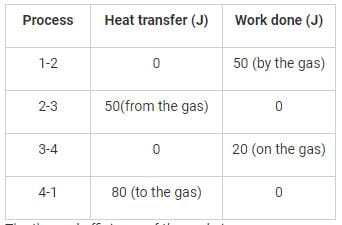The thermal efficiency of the cycle is

Solution:

Thermal efficiency is defined as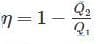Where Q2 is the heat rejected from the system and Qt is the heat added to the system.
Here Q2 = 50 J
Q1 = 80 J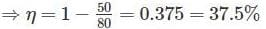QUESTION: 2

### A heat reservoir is maintained at 927 °C. If the ambient temperature is 27 °C, the availability of heat from the reservoir is limited to

Solution:

Availability is the maximum useful work that can be extracted from the system working between two reservoirs.

Availability of Heat =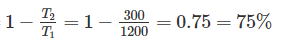QUESTION: 3

### Which of the following devices complies with the Clausius statement of the second law of thermodynamics?

Solution:

Clausius statement: No device can operate on a cycle and produce effect that is solely the heat transfer from a lower-temperature body to a higher-temperature body.

Clausius statement is related to refrigerator and heat pump whereas gas turbines works on Brayton Cycle.

QUESTION: 4

Two reversible engines are connected in series between a heat source and a sink. The efficiencies of these engines are 60% and 50%, respectively. If these two engines are replaced by a single reversible engine, the efficiency of this engine will be

Solution: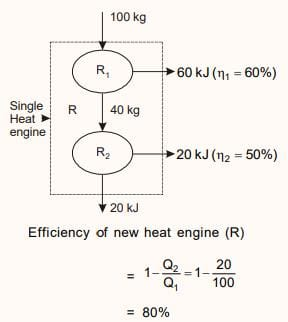QUESTION: 5

The entropy of water at 0 K is assumed to be

Solution:

Absolute zero (0 K) is a state at which the enthalpy and entropy of a system reach their minimum value, taken as 0.

In mathematical term,

S = k ln W

Where, S is entropy

k is Boltzmann constant

W is thermodynamic probability

When W = 1, it represents the greatest order, S = 0. This occurs only at T = 0 K.

This statement is also known as Third law of thermodynamics.

QUESTION: 6

The property of a working system which changes as the heat is supplied to the working fluid in a reversible manner is known as ________.

Solution:

Entropy is a property which is a measure of energy dispersion in a system or the irreversibility. Entropy transfer is associated with heat transfer. If the heat is added to the system, then its entropy increases and if heat is lost from the system, its entropy decreases.

QUESTION: 7

Two reversible heat engines operating between temperatures 2000 K and T K and T K and 500 K respectively. What is the intermediate temperature, if the efficiency of both the cycles is same?

Solution: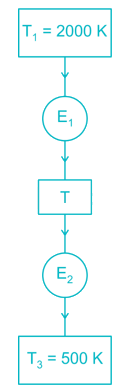Efficiency of first engine (E1),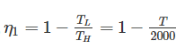Efficiency of second engine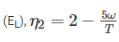As efficiency of both engine is same,

So, η1 = η2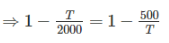⇒ T2 = 2000 × 50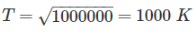Short Trick:

When efficiency of both engines are equal, then intermediate temperature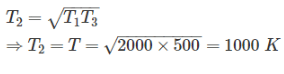QUESTION: 8

A Carnot heat engine is working with an efficiency of 50%. If the cycle is converted into a heat pump after reversing, then what is the coefficient of performance of the heat pump?

Solution:

Efficiency of Carnot engine (ηE) = 50%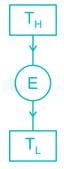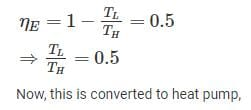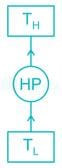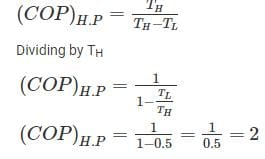Short trick:

If a Carnot heat engine which is operating between two temperature limits is converted to heat pump then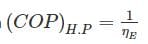QUESTION: 9

An inventor says, he has invented an engine which will reject 20% of heat absorbed from the source and the engine operates between 2000 K and 500 K. What kind of engine it is?

Solution:

T1 = 2000 K

T2 = 500 K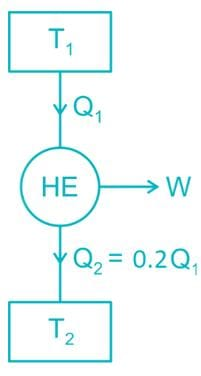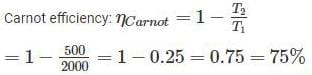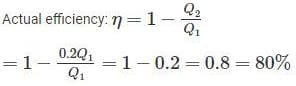Since, the actual efficiency is more than the Carnot efficiency, so this engine has an impossible cycle.

QUESTION: 10

In a cyclic process, the work done by the system is 20 kJ, -30 kJ, -5 kJ and 10 kJ. What is the net heat (kJ) for the cyclic process?

Solution:

dQ = dW + ΔU (As per first law)

For the cyclic process,

ΔU = 0

∴∫dW=∫dQ∴∫dW=∫dQ

∴ W = 20 - 30 - 5 + 10

= -5 kJ

QUESTION: 11

The heat rejection takes place in Carnot cycle at _______.

Solution:

A Carnot cycle consists of the four processes:

3. Reversible isothermal heat rejection
4. Isentropic compression of the gas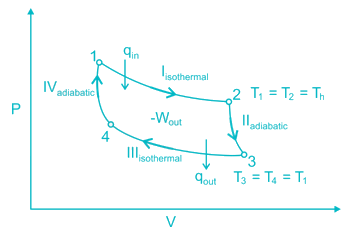QUESTION: 12

The triple point on a T-s diagram is ________.

Solution:

The pressure and temperature at which three phases of a pure substance coexist is called triple point. The triple point is merely the point of intersection of the sublimation and vaporization curves. It has been found that on a ‘p-T’ diagram the triple point is represented by a point and on a ‘p-v’ and T-s diagram it is a line, and on a ‘u-v’ diagram it is a triangle. In the case of ordinary water, the triple point is at a pressure of 4.58 mm Hg and a temperature of 0.01OC.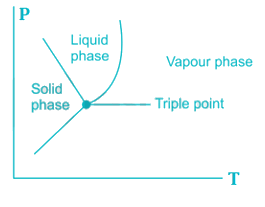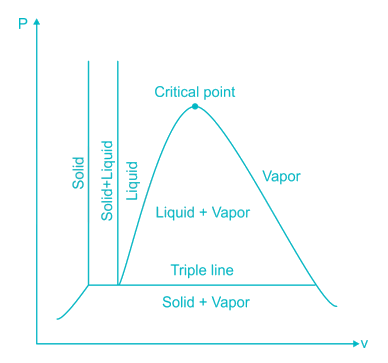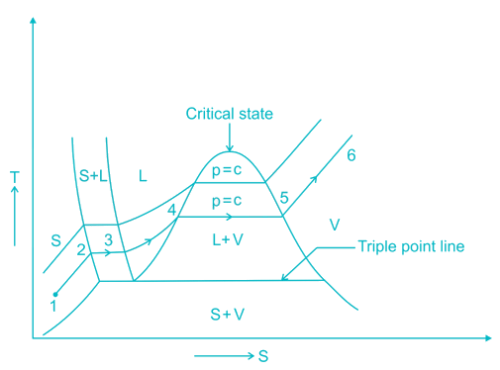QUESTION: 13

Which gas can attain the highest efficiency for the same compression rise?

Solution:

γmonoatomic> γdiatomic> γtriatomic

As efficiency is directly proportional to the ratio of specific heat (γ), so monoatomic will have the highest efficiency.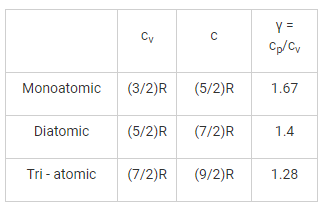QUESTION: 14

A tank containing air is stirred by a paddle wheel. The work input to the paddle wheel is 9000 kJ and heat transferred to the surroundings from the tank is 3000 kJ. The external work done by the system is:

Solution:

First law of thermodynamics:

δQ=ΔU+δWδQ=ΔU+δW

(-3000) = ΔU + (-9000)

ΔU = 6000 kJ

As there is 9000 kJ work done on the system, no work will be done by the system. Energy will be stored in the system as internal energy.

QUESTION: 15

If pressure at any point in the liquid approaches the vapor pressure, liquid starts vaporising and creates pockets or bubbles of dissolved gases and vapours. This phenomenon is ________.

Solution:

Explanation : Cavitation is a phenomenon in which rapid changes of pressure in a liquid lead to the formation of small vapor-filled cavities in places where the pressure is relatively low.

When subjected to higher pressure, these cavities, called "bubbles" or "voids", collapse and can generate a shock wave that is strong very close to the bubble, but rapidly weakens as it propagates away from the bubble.

Therefore, If pressure at any point in the liquid approaches the vapor pressure, liquid starts vaporising and creates pockets or bubbles of dissolved gases and vapours. This phenomenon is Cavitation.

QUESTION: 16

Two gases A and B with their molecular weights 28 and 44 respectively, expand at constant pressures through the same temperature range. The ratio of quantity of work done by the two gases (A : B) is ________.

Solution:

Work done, W = P2V2 - P1V1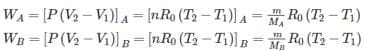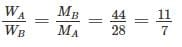QUESTION: 17

A series of operations, which takes place in a certain order and restore the initial conditions at the end, is known as

Solution:

Explanation : A series of operations, which takes place in a certain order and restores the initial conditions at the end, is known as thermodynamic cycle.

QUESTION: 18

Specific heat of a gas, Cp = Cv, at

Solution:

The specific heat of solids and liquids and the specific heats (both at constant volume and constant pressure) of ideal gases are essentially functions of temperature only. At T = 0K i.e, at the absolute zero thermodynamic temperature one should have the values of specific heats as zero putting T = 0K in the expressions for specific heats.

This strictly means at an absolute temperature of 0K we would need no energy at all to raise the temperature of unit mass of a substance by unit degree rise in temperature. But as soon as the temperature rises by even an infinitesimal amount, we have some value of the specific heat and it increases with temperature and hence more and more energy would be needed to achieve a similar rise in temperature as temperature increases.

QUESTION: 19

Joule-Thompson coefficient for an ideal gas is

Solution:

Joule Thomson Coefficient:-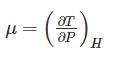For an ideal gas, μ = 0, because ideal gases neither warm not cool upon being expanded at constant enthalpy.

QUESTION: 20

A thermometer works on the principle of

Solution:

A thermometer works on the principle of Zeroth law of Thermodynamics.

According to Zeroth Law of thermodynamics, if two bodies are in thermal equilibrium with a third body then the two bodies are also in thermal equilibrium with each other.

Thermometer are based on the principle of finding the temperature by measuring the thermometric property.

The measurement of temperature is performed by the thermometer by using mercury, which - when heated - expands, and contracts in response to a fall in temperature. This causes the length of the liquid column to be longer or shorter depending on the temperature.Use Code STAYHOME200 and get INR 200 additional OFF Use Coupon Code